Courses

# 28 Year NEET Questions: Motion in a Plane- 2

## 30 Questions MCQ Test Physics 31 Years NEET Chapterwise Solved Papers | 28 Year NEET Questions: Motion in a Plane- 2

Description
This mock test of 28 Year NEET Questions: Motion in a Plane- 2 for NEET helps you for every NEET entrance exam. This contains 30 Multiple Choice Questions for NEET 28 Year NEET Questions: Motion in a Plane- 2 (mcq) to study with solutions a complete question bank. The solved questions answers in this 28 Year NEET Questions: Motion in a Plane- 2 quiz give you a good mix of easy questions and tough questions. NEET students definitely take this 28 Year NEET Questions: Motion in a Plane- 2 exercise for a better result in the exam. You can find other 28 Year NEET Questions: Motion in a Plane- 2 extra questions, long questions & short questions for NEET on EduRev as well by searching above.
QUESTION: 1

### The magnitudes of vectors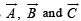are 3, 4 and 5 units respectively. If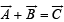, thenthe angle between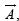and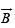is

Solution:

Given,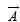= 3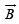= 4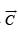= 5

We have a relation,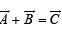Squaring both side, we get

⇒ (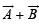) = (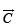)2

⇒(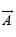)2+ (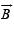)2 + 2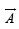Cosθ = (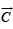)2

⇒ (3)2 + (4)2 + 2.3.4 Cosθ = (5)2

⇒ 9 + 16 + 24 Cosθ = 25

⇒ 24 Cosθ = 25

⇒ 24Cosθ = 25 - 25

⇒ θ = Cos-1 (0)

⇒ θ = 90°

i.e π/2

QUESTION: 2

### The angle between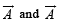is θ.  The value of the triple product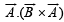is 

Solution:

Note that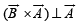. Hence their dot product is zero.

QUESTION: 3

### A bus is moving on a straight road towards north with a uniform speed of 50 km/hour turns through 90°. If the speed remains unchanged after turning, the increase in the velocity of bus in the turning process is 

Solution: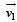= 50 km h-1 due North;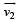= 50 km h-1 due West. Angle between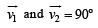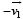= 50 km h-1  due South

∴ Change in velocity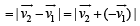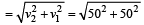= 70.7 km/h

The direction of this change in velocity is in South-West.

QUESTION: 4

The maximum range of a gun of horizontal terrain is 16 km. If g = 10 ms–2, then muzzle velocity of a shell must be 

Solution: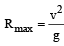= 16000 [16km = 16000m]

or  v = (16000g )½ = (16000 x10)½

= 400 ms –1

QUESTION: 5

Two bodies of same mass are projected with the same velocity at an angle 30° and 60° respectively. The ratio of their horizontal ranges will be

Solution:

Horizontal range is same when angle of projection is θ or (90° – θ).

QUESTION: 6

An electricfan has blades of length 30cm measured from the axis of rotation. If the fan is rotating at 120 rpm, the acceleration of a point on the tip of the blade is 

Solution: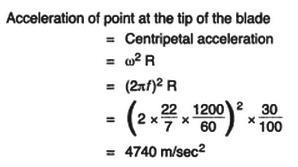QUESTION: 7

When milk is churned, cream gets separated due to

Solution:

Cream gets separated from a churned milk due to centrifugal force.

QUESTION: 8

The resultant of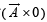will be equal to 

Solution:

When a vector is multiplied with a scalar, the result is a vector.

QUESTION: 9

If a body  A  of mass  M  is thrown with velocity v  at an angle of 30° to the horizontal and another body  B  of the  same mass is thrown with the same speed at an angle of 60° to the horizontal, the ratio of horizontal range of A to B will be 

Solution:

Horizontal range is same wh en an gle of projection with the horizonatal is θ and (90° – θ).

QUESTION: 10

A boat is sent across a river with a velocity of 8 km h–1. If the resultant velocity of boat is 10 km h–1 , then the velocity of the river is 

Solution: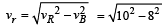= 6 km h –111.

QUESTION: 11

A body constrained to move in y-direction, is subjected to a force given  by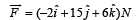. What is the workdone by this force in moving the body through a distance of 10m along y-axis ? 

Solution:

Since displacement is along the y-direction, hence displacement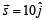Work done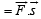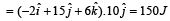QUESTION: 12

When a body moves with a constant speed along a circle

Solution:

On circular motion, the force acts along the radius and displacement at a location is perpendicular to the radius i.e., θ = 90° As work done =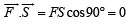QUESTION: 13

Th e position vector of a par ticle is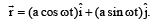. The velocity of the particle is 

Solution: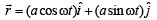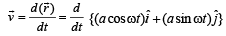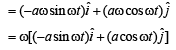Slope of position vector =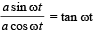& slope of velocity vector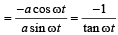∴  velocity is perpendicular to the displacement.

QUESTION: 14

Which of the following is not a vector quantity?

Solution:

A vector quantity has both magnitude and direction. In the given options, speed has only magnitude, therefore, it is non- vector or scalar quantity.

QUESTION: 15

A bullet is fired from a gun with a speed of 1000 m/s in order to hit a target 100 m away. At what height above the target should the gun be aimed? (The resistance of air is negligible and g = 10 m/s2) 

Solution:

Speed of the bullet (v) = 1000 m/s and horizontal distance of the target (s) = 100 m.
Time taken to cover the horizontal distance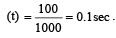During this time, the bullet will fall down vertically due to gravitational acceleration.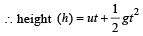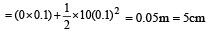QUESTION: 16

A body is whirled in a horizontal circle of radius 20 cm. It has an angular velocity of 10 rad/s.What is its linear velocity at any point on circular path

Solution:

Radius of circular path = 20 cm =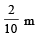Angular speed of body = 10 rad/s

Linear velocity = radius × Angular speed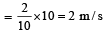QUESTION: 17

A ball whose kinetic energy is E, is thrown at an angle of 45º with the horizontal. Its kinetic energy at the highest point of its flight will be 

Solution:

Kinetic energy is E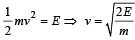Velocity at highest point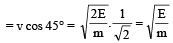[∴ at the highest point there is only horizontal velocity]

K.E. at highest point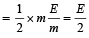QUESTION: 18

Find the torque of a force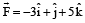acting at the poin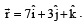. 

Solution: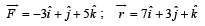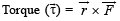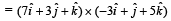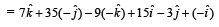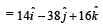QUESTION: 19

Two particles A an d B are con nected by a rigid rod AB. The rod slides along perpendicular rails as shown here. The velocity of A to the left is 10 m/s. What is the velocity of B when angle α = 60º?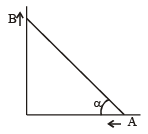Solution:

Let after 1 sec angle become 60°. When the end A moves by 10 m left, the end B moves upward by BB' =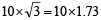= 17.3 m/s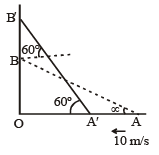QUESTION: 20

A ball of mass 0.25 kg attached to the end of a string of length 1.96 m is moving in a horizontal circle. The string will break if the tension is more than 25 N. What is the maximum speed with which the ball can be moved? 

Solution: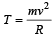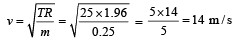QUESTION: 21

A 500 kg car takes a round turn of radius 50 m with a velocity of 36 km/h. The centripetal force is

Solution:

V =36km/h=10m/s

Centripetal force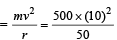= 1000 N

QUESTION: 22

What is the linear velocity if angular velocity vect or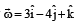and position vector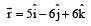Solution:

As we know that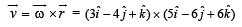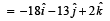QUESTION: 23

The angle between two vectors of magnitude 12 and 18 units when their resultant is 24 units, is

Solution:

We know that, R2 = A2 + B2 + 2 AB cosθ
(24)2 = (12)2 + (18)2 + 2(12)(18) cos θ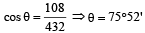QUESTION: 24

A body of mass 0.4 kg is whi rled in a vertical circle making 2 rev/sec. If the radius of the circle is 1.2 m, then tension in the string when the body is at th e top of the circle, is 

Solution:

Given : Mass (m) = 0.4 kg Its frequency (n) = 2 rev/sec Radius (r) =1.2 m.

We know that linear velocity of the body (v) = ωr = (2πn)r

= 2 × 3.14 × 1.2 × 2 = 15.08 m/s.

Therefore, tension in the string when the body is at the top of the circle (T)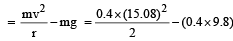= 45.78 - 3.92 = 41.56 N

QUESTION: 25

A stone tied with a string, is rotated in a vertical circle. The minimum speed with which the string has to be rotated 

Solution:

Minimum speed with which the string is rotating in a vertical circle (v) =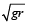The minimum speed of stone is independent of mass of stone.

QUESTION: 26

A person swims in a river aiming to reach exactly opposite point on the bank of a river. His speed of swimming is 0.5 m/s at an angle 120º with the direction of flow of water. The speed of water in stream is 

Solution: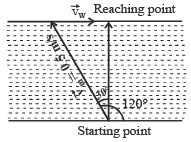Velocity of person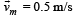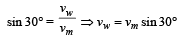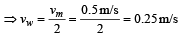QUESTION: 27

If a unit vector is represented by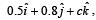, the value of c is 

Solution: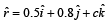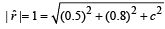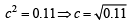QUESTION: 28

Two projectiles ar e fir ed from t he same point with the same speed at angles of projection 60º and 30º respectively. Which one of the following is true? 

Solution:

Given, u1 = u2 = u, θ1 = 60º, θ2 = 30º In 1st case, we know that range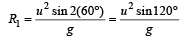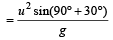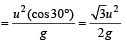In 2nd case, when θ2 = 30º, then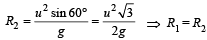[we get same value of ranges].

QUESTION: 29

A small sphere is attach ed to a cord and rotates in a vertical circle about a point O. If the average speed of the sphere is increased, the cord is most likely to break at the orientation when the mass is at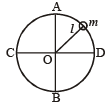Solution:

In the case of a body describing a vertical circle,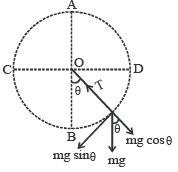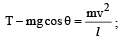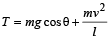Tension is maximum when cosθ = +1 and velocity is maximum Both conditions are satisfied at θ = 0º (i.e. at lowest point B]

QUESTION: 30

A particle moves with a velocity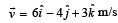under the in fluence of a constant force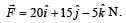The instantaneous power applied to the particle is 

Solution: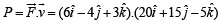= 6 × 20 – 4 × 15 –3 ×5 = 45 J/s